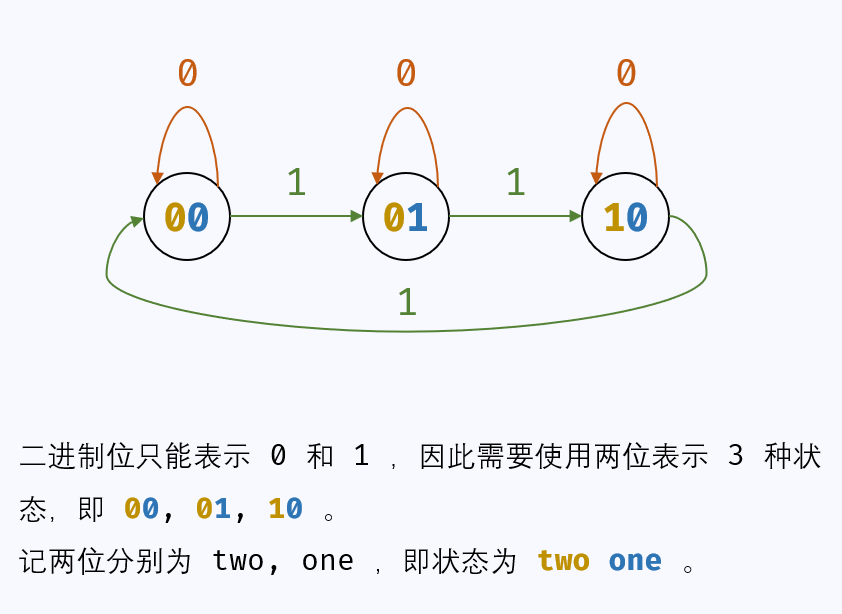# 1、找出单独出现的数字

## 1.2、算法

### 1.2.1、算法1：集合

/**
* 算法1：ArrayList
*/
public int singleNumber1(int[] nums) {
List<Integer> list = new ArrayList<>();
for (int i = 0; i < nums.length; i++) {
if (list.contains(nums[i])) {
list.remove((Integer) nums[i]);
} else {
}
}
return list.get(0);
}


### 1.2.3、算法2：HashMap

/**
* 算法2：HashMap
*/
public int singleNumber2(int[] nums) {
//map收集每个数字出现的个数
Map<Integer, Integer> map = new HashMap<>();
for (int i = 0; i < nums.length; i++) {
map.put(nums[i], map.getOrDefault(nums[i], 0) +1);
}
//找出数量为1的个数
for (Integer key : map.keySet()){
if (map.get(key) == 1){
return key;
}
}
return 0 ;
}


### 1.2.3、算法3：位运算

/**
* 算法2：官方
*/
public int singleNumber(int[] nums) {
int result = 0;
for (int num : nums) {
result = result ^ num;
}
return result;
}


## 1.3、测试

 @Test
public void test(){
int[] nums = {4,1,2,1,2};
System.out.println(singleNumber1(nums));
System.out.println(singleNumber(nums));
}


# 2、找出单独出现的数字

输入: [2,2,3,2]



输入: [0,1,0,1,0,1,99]



## 2.1、解题思路

### 2.1.2、算法2解题思路

1、出现0次为0，出现1次为1，出现2次的值无所谓(因为题目中说明了，肯定是3次)，但是出现3次就又回到0，也就是说，我们一共需要记录3种状态：0次，1次，2次，之后次数都是这三种状态的循环

2、记录两个状态需要的是一位二进制0/1，那么记录三种状态需要的是至少两位二进制，可以是00, 01, 10, 11，这里我们只需要选其中任意三个状态即可（因为到了第三次直接就变成00了），例如：00，01，10，分别代表0次1次2次。

3、那么对于输入数字的每一位二进制位，都可以用这三种状态表示。如果再输入一个数字，对于每一位上，我们的操作可以化为：

​ 新输入的是0（即00），三种状态都维持不变，00→00,01→01,10→10

​ 新输入的是1（即01），00→01,01→10,10→00if two == 0:
if n == 0:
one = one
if n == 1:
one = ~one
if two == 1:
one = 0


if two == 0:
one = one ^ n
if two == 1:
one = 0


one = one ^ n & ~two


two = two ^ n & ~one


/**
* 算法2：官方
* 异或运算：x ^ 0 = x​ ， x ^ 1 = ~x
* 与运算：  x & 0 = 0 ， x & 1 = x
* 仅当 seen_twice 未变时，改变 seen_once。
* 仅当 seen_once 未变时，改变seen_twice。
* 位掩码 seen_once 仅保留出现一次的数字，不保留出现三次的数字。
*/
public int singleNumber(int[] nums) {
//初始就是00
int seenOnce = 0; //后一位状态
int seenTwice = 0; //前一位状态
for (int num : nums) {
seenOnce = (seenOnce ^ num) & ~seenTwice;
seenTwice = (seenTwice ^ num) & ~seenOnce;
}
//结尾状态为肯定是01，所以取后面的这个
return seenOnce;
}


## 2.2、算法

### 2.2.1、算法1：HashMap

/**
* 算法1：HashMap
*/
public int singleNumber1(int[] nums) {
//map收集每个数字出现的个数
Map<Integer, Integer> map = new HashMap<>();
for (int i = 0; i < nums.length; i++) {
map.put(nums[i], map.getOrDefault(nums[i], 0) +1);
}
//找出数量为1的个数
for (Integer key : map.keySet()){
if (map.get(key) == 1){
return key;
}
}
return 0 ;
}


### 2.2.1、算法2：位运算

/**
* 算法2：官方
* 异或运算：x ^ 0 = x​ ， x ^ 1 = ~x
* 与运算：  x & 0 = 0 ， x & 1 = x
* 仅当 seen_twice 未变时，改变 seen_once。
* 仅当 seen_once 未变时，改变seen_twice。
* 位掩码 seen_once 仅保留出现一次的数字，不保留出现三次的数字。
*/
public int singleNumber(int[] nums) {
//初始就是00
int seenOnce = 0; //后一位状态
int seenTwice = 0; //前一位状态
for (int num : nums) {
seenOnce = (seenOnce ^ num) & ~seenTwice;
seenTwice = (seenTwice ^ num) & ~seenOnce;
}
//结尾状态为肯定是01，所以取后面的这个
return seenOnce;
}


## 2.3、测试

@Test
public void test(){
int[] nums = {4,1,2,1,2};
System.out.println(singleNumber1(nums));
System.out.println(singleNumber(nums));
}


# 3、找出单独出现的数字

输入: [1,2,1,3,2,5]



## 3.2、算法

### 3.2.1、算法1：HashMap

/**
* 算法1：
*/
public int[] singleNumber(int[] nums) {
int[] array = new int;
int idx = 0 ;
Map<Integer, Integer> map = new HashMap<>();
for (int i = 0; i < nums.length; i++) {
map.put(nums[i], map.getOrDefault(nums[i], 0) + 1);
}
for (Integer key : map.keySet()){
if (map.get(key) == 1){
array[idx++] = key;
}
}
return array;
}


## 3.3、测试

@Test
public void test(){
int[] nums = {4,1,2,1,2};
System.out.println(singleNumber(nums));
}